# tsfresh.feature_extraction package¶

## tsfresh.feature_extraction.extraction module¶

This module contains the main function to interact with tsfresh: extract features

tsfresh.feature_extraction.extraction.extract_features(timeseries_container, default_fc_parameters=None, kind_to_fc_parameters=None, column_id=None, column_sort=None, column_kind=None, column_value=None, chunksize=None, n_jobs=2, show_warnings=False, disable_progressbar=False, impute_function=None, profile=False, profiling_filename='profile.txt', profiling_sorting='cumulative')[source]

Extract features from

or

In both cases a pandas.DataFrame with the calculated features will be returned.

For a list of all the calculated time series features, please see the ComprehensiveFCParameters class, which is used to control which features with which parameters are calculated.

For a detailed explanation of the different parameters and data formats please see Data Formats.

Examples

>>> from tsfresh.examples import load_robot_execution_failures
>>> from tsfresh import extract_features
>>> X = extract_features(df, column_id='id', column_sort='time')

Parameters: timeseries_container (pandas.DataFrame or dict) – The pandas.DataFrame with the time series to compute the features for, or a dictionary of pandas.DataFrames. default_fc_parameters (dict) – mapping from feature calculator names to parameters. Only those names which are keys in this dict will be calculated. See the class:ComprehensiveFCParameters for more information. kind_to_fc_parameters (dict) – mapping from kind names to objects of the same type as the ones for default_fc_parameters. If you put a kind as a key here, the fc_parameters object (which is the value), will be used instead of the default_fc_parameters. column_id (str) – The name of the id column to group by. column_sort (str) – The name of the sort column. column_kind (str) – The name of the column keeping record on the kind of the value. column_value (str) – The name for the column keeping the value itself. n_jobs (int) – The number of processes to use for parallelization. If zero, no parallelization is used. chunksize (None or int) – The size of one chunk for the parallelisation disable_progressbar (bool) – Do not show a progressbar while doing the calculation. impute_function (None or callable) – None, if no imputing should happen or the function to call for imputing. profile (bool) – Turn on profiling during feature extraction profiling_sorting (basestring) – How to sort the profiling results (see the documentation of the profiling package for more information) profiling_filename (basestring) – Where to save the profiling results. show_warnings: Show warnings during the feature extraction (needed for debugging of calculators). The (maybe imputed) DataFrame containing extracted features. pandas.DataFrame

## tsfresh.feature_extraction.feature_calculators module¶

This module contains the feature calculators that take time series as input and calculate the values of the feature. There are two types of features:

1. feature calculators which calculate a single number (simple)
2. feature calculators which calculate a bunch of features for a list of parameters at once, to use e.g. cached results (combiner). They return a list of (key, value) pairs for each input parameter.

They are specified using the “fctype” parameter of each feature calculator, which is added using the set_property function. Only functions in this python module, which have a parameter called “fctype” are seen by tsfresh as a feature calculator. Others will not be calculated.

tsfresh.feature_extraction.feature_calculators.abs_energy(x)[source]

Returns the absolute energy of the time series which is the sum over the squared values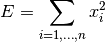Parameters: x (pandas.Series) – the time series to calculate the feature of the value of this feature float

This function is of type: simple

tsfresh.feature_extraction.feature_calculators.absolute_sum_of_changes(x)[source]

Returns the sum over the absolute value of consecutive changes in the series x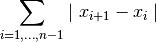Parameters: x (pandas.Series) – the time series to calculate the feature of the value of this feature float

This function is of type: simple

tsfresh.feature_extraction.feature_calculators.agg_autocorrelation(x, param)[source]

Calculates the value of an aggregation function f_agg (e.g. var or mean) of the autocorrelation (Compare to http://en.wikipedia.org/wiki/Autocorrelation#Estimation), taken over different all possible lags (1 to length of x)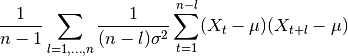where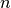is the length of the time series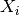,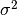its variance and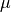its mean.

Parameters: x (pandas.Series) – the time series to calculate the feature of param (list) – contains dictionaries {“attr”: x} with x str, name of a numpy function (e.g. mean, var, std, median), the name of the aggregator function that is applied to the autocorrelations the value of this feature float

This function is of type: combiner

tsfresh.feature_extraction.feature_calculators.agg_linear_trend(x, param)[source]

Calculates a linear least-squares regression for values of the time series that were aggregated over chunks versus the sequence from 0 up to the number of chunks minus one.

This feature assumes the signal to be uniformly sampled. It will not use the time stamps to fit the model.

The parameters attr controls which of the characteristics are returned. Possible extracted attributes are “pvalue”, “rvalue”, “intercept”, “slope”, “stderr”, see the documentation of linregress for more information.

The chunksize is regulated by “chunk_len”. It specifies how many time series values are in each chunk.

Further, the aggregation function is controlled by “f_agg”, which can use “max”, “min” or , “mean”, “median”

Parameters: x (pandas.Series) – the time series to calculate the feature of param (list) – contains dictionaries {“attr”: x, “chunk_len”: l, “f_agg”: f} with x, f an string and l an int the different feature values pandas.Series

This function is of type: combiner

tsfresh.feature_extraction.feature_calculators.approximate_entropy(x, m, r)[source]

Implements a vectorized Approximate entropy algorithm.

For short time-series this method is highly dependent on the parameters, but should be stable for N > 2000, see:

Yentes et al. (2012) - The Appropriate Use of Approximate Entropy and Sample Entropy with Short Data Sets

Other shortcomings and alternatives discussed in:

Richman & Moorman (2000) - Physiological time-series analysis using approximate entropy and sample entropy
Parameters: x (pandas.Series) – the time series to calculate the feature of m (int) – Length of compared run of data r (float) – Filtering level, must be positive Approximate entropy float

This function is of type: simple

tsfresh.feature_extraction.feature_calculators.ar_coefficient(x, param)[source]

This feature calculator fits the unconditional maximum likelihood of an autoregressive AR(k) process. The k parameter is the maximum lag of the process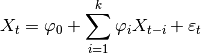For the configurations from param which should contain the maxlag “k” and such an AR process is calculated. Then the coefficients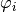whose indexcontained from “coeff” are returned.

Parameters: x (pandas.Series) – the time series to calculate the feature of param (list) – contains dictionaries {“coeff”: x, “k”: y} with x,y int the different feature values pandas.Series

This function is of type: combiner

tsfresh.feature_extraction.feature_calculators.augmented_dickey_fuller(x, param)[source]

The Augmented Dickey-Fuller test is a hypothesis test which checks whether a unit root is present in a time series sample. This feature calculator returns the value of the respective test statistic.

See the statsmodels implementation for references and more details.

Parameters: x (pandas.Series) – the time series to calculate the feature of param (list) – contains dictionaries {“attr”: x} with x str, either “teststat”, “pvalue” or “usedlag” the value of this feature float

This function is of type: combiner

tsfresh.feature_extraction.feature_calculators.autocorrelation(x, lag)[source]

Calculates the autocorrelation of the specified lag, according to the formula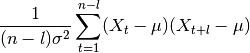whereis the length of the time series,its variance andits mean. l denotes the lag.

References

Parameters: x (pandas.Series) – the time series to calculate the feature of lag (int) – the lag the value of this feature float

This function is of type: simple

tsfresh.feature_extraction.feature_calculators.binned_entropy(x, max_bins)[source]

First bins the values of x into max_bins equidistant bins. Then calculates the value of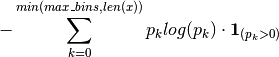where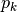is the percentage of samples in bin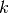.

Parameters: x (pandas.Series) – the time series to calculate the feature of max_bins (int) – the maximal number of bins the value of this feature float

This function is of type: simple

tsfresh.feature_extraction.feature_calculators.c3(x, lag)[source]

This function calculates the value of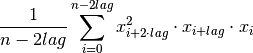which is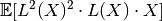where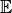is the mean and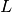is the lag operator. It was proposed in  as a measure of non linearity in the time series.

References

 Schreiber, T. and Schmitz, A. (1997).
Discrimination power of measures for nonlinearity in a time series
PHYSICAL REVIEW E, VOLUME 55, NUMBER 5
Parameters: x (pandas.Series) – the time series to calculate the feature of lag (int) – the lag that should be used in the calculation of the feature the value of this feature float

This function is of type: simple

tsfresh.feature_extraction.feature_calculators.change_quantiles(x, ql, qh, isabs, f_agg)[source]

First fixes a corridor given by the quantiles ql and qh of the distribution of x. Then calculates the average, absolute value of consecutive changes of the series x inside this corridor.

Think about selecting a corridor on the y-Axis and only calculating the mean of the absolute change of the time series inside this corridor.

Parameters: x (pandas.Series) – the time series to calculate the feature of ql (float) – the lower quantile of the corridor qh (float) – the higher quantile of the corridor isabs (bool) – should the absolute differences be taken? f_agg (str, name of a numpy function (e.g. mean, var, std, median)) – the aggregator function that is applied to the differences in the bin the value of this feature float

This function is of type: simple

tsfresh.feature_extraction.feature_calculators.count_above_mean(x)[source]

Returns the number of values in x that are higher than the mean of x

Parameters: x (pandas.Series) – the time series to calculate the feature of the value of this feature float

This function is of type: simple

tsfresh.feature_extraction.feature_calculators.count_below_mean(x)[source]

Returns the number of values in x that are lower than the mean of x

Parameters: x (pandas.Series) – the time series to calculate the feature of the value of this feature float

This function is of type: simple

tsfresh.feature_extraction.feature_calculators.cwt_coefficients(x, param)[source]

Calculates a Continuous wavelet transform for the Ricker wavelet, also known as the “Mexican hat wavelet” which is defined by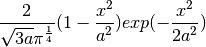where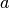is the width parameter of the wavelet function.

This feature calculator takes three different parameter: widths, coeff and w. The feature calculater takes all the different widths arrays and then calculates the cwt one time for each different width array. Then the values for the different coefficient for coeff and width w are returned. (For each dic in param one feature is returned)

Parameters: x (pandas.Series) – the time series to calculate the feature of param (list) – contains dictionaries {“widths”:x, “coeff”: y, “w”: z} with x array of int and y,z int the different feature values pandas.Series

This function is of type: combiner

tsfresh.feature_extraction.feature_calculators.energy_ratio_by_chunks(x, param)[source]

Calculates the sum of squares of chunk i out of N chunks expressed as a ratio with the sum of squares over the whole series

Takes as input parameters the number num_segments of segments to divide the series into and segment_focus which is the segment number (starting at zero) to return a feature on.

Note that the answer for num_segments=1 is a trivial “1” but we handle this scenario in case somebody calls it. Sum of the ratios should be 1.0.

Returns an error for N <= 0

Parameters: x (pandas.Series) – the time series to calculate the feature of param – contains dictionaries {“num_segments”: N, “segment_focus”: i} with N, i both ints the feature values list of tuples (index, data)

This function is of type: combiner

tsfresh.feature_extraction.feature_calculators.fft_coefficient(x, param)[source]

Calculates the fourier coefficients of the one-dimensional discrete Fourier Transform for real input by fast fourier transformation algorithm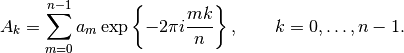The resulting coefficients will be complex, this feature calculator can return the real part (attr==”real”), the imaginary part (attr==”imag), the absolute value (attr=”“abs) and the angle in degrees (attr==”angle).

Parameters: x (pandas.Series) – the time series to calculate the feature of param (list) – contains dictionaries {“coeff”: x, “attr”: s} with x int and x >= 0, s str and in [“real”, “imag”, “abs”, “angle”] the different feature values pandas.Series

This function is of type: combiner

tsfresh.feature_extraction.feature_calculators.first_location_of_maximum(x)[source]

Returns the first location of the maximum value of x. The position is calculated relatively to the length of x.

Parameters: x (pandas.Series) – the time series to calculate the feature of the value of this feature float

This function is of type: simple

tsfresh.feature_extraction.feature_calculators.first_location_of_minimum(x)[source]

Returns the first location of the minimal value of x. The position is calculated relatively to the length of x.

Parameters: x (pandas.Series) – the time series to calculate the feature of the value of this feature float

This function is of type: simple

tsfresh.feature_extraction.feature_calculators.friedrich_coefficients(x, param)[source]

Coefficients of polynomial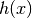, which has been fitted to the deterministic dynamics of Langevin model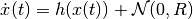as described by .

For short time-series this method is highly dependent on the parameters.

References

 Friedrich et al. (2000): Physics Letters A 271, p. 217-222
Extracting model equations from experimental data
Parameters: x (pandas.Series) – the time series to calculate the feature of c (str) – the time series name param (list) – contains dictionaries {“coeff”: x} with x int and x >= 0 the different feature values pandas.Series

This function is of type: combiner

tsfresh.feature_extraction.feature_calculators.has_duplicate(x)[source]

Checks if any value in x occurs more than once

Parameters: x (pandas.Series) – the time series to calculate the feature of the value of this feature bool

This function is of type: simple

tsfresh.feature_extraction.feature_calculators.has_duplicate_max(x)[source]

Checks if the maximum value of x is observed more than once

Parameters: x (pandas.Series) – the time series to calculate the feature of the value of this feature bool

This function is of type: simple

tsfresh.feature_extraction.feature_calculators.has_duplicate_min(x)[source]

Checks if the minimal value of x is observed more than once

Parameters: x (pandas.Series) – the time series to calculate the feature of the value of this feature bool

This function is of type: simple

tsfresh.feature_extraction.feature_calculators.index_mass_quantile(x, param)[source]

Those apply features calculate the relative index i where q% of the mass of the time series x lie left of i. For example for q = 50% this feature calculator will return the mass center of the time series

Parameters: x (pandas.Series) – the time series to calculate the feature of param (list) – contains dictionaries {“q”: x} with x float the different feature values pandas.Series

This function is of type: combiner

tsfresh.feature_extraction.feature_calculators.kurtosis(x)[source]

Returns the kurtosis of x (calculated with the adjusted Fisher-Pearson standardized moment coefficient G2).

Parameters: x (pandas.Series) – the time series to calculate the feature of the value of this feature float

This function is of type: simple

tsfresh.feature_extraction.feature_calculators.large_standard_deviation(x, r)[source]

Boolean variable denoting if the standard dev of x is higher than ‘r’ times the range = difference between max and min of x. Hence it checks if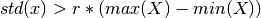According to a rule of the thumb, the standard deviation should be a forth of the range of the values.

Parameters: x (pandas.Series) – the time series to calculate the feature of r (float) – the percentage of the range to compare with the value of this feature bool

This function is of type: simple

tsfresh.feature_extraction.feature_calculators.last_location_of_maximum(x)[source]

Returns the relative last location of the maximum value of x. The position is calculated relatively to the length of x.

Parameters: x (pandas.Series) – the time series to calculate the feature of the value of this feature float

This function is of type: simple

tsfresh.feature_extraction.feature_calculators.last_location_of_minimum(x)[source]

Returns the last location of the minimal value of x. The position is calculated relatively to the length of x.

Parameters: x (pandas.Series) – the time series to calculate the feature of the value of this feature float

This function is of type: simple

tsfresh.feature_extraction.feature_calculators.length(x)[source]

Returns the length of x

Parameters: x (pandas.Series) – the time series to calculate the feature of the value of this feature int

This function is of type: simple

tsfresh.feature_extraction.feature_calculators.linear_trend(x, param)[source]

Calculate a linear least-squares regression for the values of the time series versus the sequence from 0 to length of the time series minus one. This feature assumes the signal to be uniformly sampled. It will not use the time stamps to fit the model. The parameters control which of the characteristics are returned.

Possible extracted attributes are “pvalue”, “rvalue”, “intercept”, “slope”, “stderr”, see the documentation of linregress for more information.

Parameters: x (pandas.Series) – the time series to calculate the feature of param (list) – contains dictionaries {“attr”: x} with x an string, the attribute name of the regression model the different feature values pandas.Series

This function is of type: combiner

tsfresh.feature_extraction.feature_calculators.longest_strike_above_mean(x)[source]

Returns the length of the longest consecutive subsequence in x that is bigger than the mean of x

Parameters: x (pandas.Series) – the time series to calculate the feature of the value of this feature float

This function is of type: simple

tsfresh.feature_extraction.feature_calculators.longest_strike_below_mean(x)[source]

Returns the length of the longest consecutive subsequence in x that is smaller than the mean of x

Parameters: x (pandas.Series) – the time series to calculate the feature of the value of this feature float

This function is of type: simple

tsfresh.feature_extraction.feature_calculators.max_langevin_fixed_point(x, r, m)[source]

Largest fixed point of dynamics :math:argmax_x {h(x)=0} estimated from polynomial, which has been fitted to the deterministic dynamics of Langevin model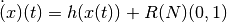as described by

Friedrich et al. (2000): Physics Letters A 271, p. 217-222 Extracting model equations from experimental data

For short time-series this method is highly dependent on the parameters.

Parameters: x (pandas.Series) – the time series to calculate the feature of m (int) – order of polynom to fit for estimating fixed points of dynamics r (float) – number of quantils to use for averaging Largest fixed point of deterministic dynamics float

This function is of type: simple

tsfresh.feature_extraction.feature_calculators.maximum(x)[source]

Calculates the highest value of the time series x.

Parameters: x (pandas.Series) – the time series to calculate the feature of the value of this feature float

This function is of type: simple

tsfresh.feature_extraction.feature_calculators.mean(x)[source]

Returns the mean of x

Parameters: x (pandas.Series) – the time series to calculate the feature of the value of this feature float

This function is of type: simple

tsfresh.feature_extraction.feature_calculators.mean_abs_change(x)[source]

Returns the mean over the absolute differences between subsequent time series values which is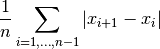Parameters: x (pandas.Series) – the time series to calculate the feature of the value of this feature float

This function is of type: simple

tsfresh.feature_extraction.feature_calculators.mean_change(x)[source]

Returns the mean over the absolute differences between subsequent time series values which is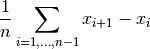Parameters: x (pandas.Series) – the time series to calculate the feature of the value of this feature float

This function is of type: simple

tsfresh.feature_extraction.feature_calculators.mean_second_derivate_central(x)[source]

Returns the mean value of a central approximation of the second derivative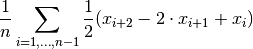Parameters: x (pandas.Series) – the time series to calculate the feature of the value of this feature float

This function is of type: simple

tsfresh.feature_extraction.feature_calculators.median(x)[source]

Returns the median of x

Parameters: x (pandas.Series) – the time series to calculate the feature of the value of this feature float

This function is of type: simple

tsfresh.feature_extraction.feature_calculators.minimum(x)[source]

Calculates the lowest value of the time series x.

Parameters: x (pandas.Series) – the time series to calculate the feature of the value of this feature float

This function is of type: simple

tsfresh.feature_extraction.feature_calculators.number_crossing_m(x, m)[source]

Calculates the number of crossings of x on m. A crossing is defined as two sequential values where the first value is lower than m and the next is greater, or vice-versa. If you set m to zero, you will get the number of zero crossings.

Parameters: x (pandas.Series) – the time series to calculate the feature of m (float) – the threshold for the crossing the value of this feature float

This function is of type: simple

tsfresh.feature_extraction.feature_calculators.number_cwt_peaks(x, n)[source]

This feature calculator searches for different peaks in x. To do so, x is smoothed by a ricker wavelet and for widths ranging from 1 to n. This feature calculator returns the number of peaks that occur at enough width scales and with sufficiently high Signal-to-Noise-Ratio (SNR)

Parameters: x (pandas.Series) – the time series to calculate the feature of n (int) – maximum width to consider the value of this feature int

This function is of type: simple

tsfresh.feature_extraction.feature_calculators.number_peaks(x, n)[source]

Calculates the number of peaks of at least support n in the time series x. A peak of support n is defined as a subsequence of x where a value occurs, which is bigger than its n neighbours to the left and to the right.

Hence in the sequence

>>> x = [3, 0, 0, 4, 0, 0, 13]


4 is a peak of support 1 and 2 because in the subsequences

>>> [0, 4, 0]
>>> [0, 0, 4, 0, 0]


4 is still the highest value. Here, 4 is not a peak of support 3 because 13 is the 3th neighbour to the right of 4 and its bigger than 4.

Parameters: x (pandas.Series) – the time series to calculate the feature of n (int) – the support of the peak the value of this feature float

This function is of type: simple

tsfresh.feature_extraction.feature_calculators.partial_autocorrelation(x, param)[source]

Calculates the value of the partial autocorrelation function at the given lag. The lag k partial autocorrelation of a time series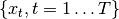equals the partial correlation of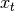and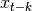, adjusted for the intermediate variables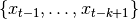(). Following , it can be defined as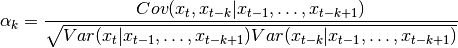with (a)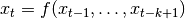and (b)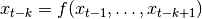being AR(k-1) models that can be fitted by OLS. Be aware that in (a), the regression is done on past values to predictwhereas in (b), future values are used to calculate the past value. It is said in  that “for an AR(p), the partial autocorrelations [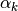] will be nonzero for k<=p and zero for k>p.” With this property, it is used to determine the lag of an AR-Process.

References

 Box, G. E., Jenkins, G. M., Reinsel, G. C., & Ljung, G. M. (2015).
Time series analysis: forecasting and control. John Wiley & Sons.
Parameters: x (pandas.Series) – the time series to calculate the feature of param (list) – contains dictionaries {“lag”: val} with int val indicating the lag to be returned the value of this feature float

This function is of type: combiner

tsfresh.feature_extraction.feature_calculators.percentage_of_reoccurring_datapoints_to_all_datapoints(x)[source]

Returns the percentage of unique values, that are present in the time series more than once.

len(different values occurring more than once) / len(different values)

This means the percentage is normalized to the number of unique values, in contrast to the percentage_of_reoccurring_values_to_all_values.

Parameters: x (pandas.Series) – the time series to calculate the feature of the value of this feature float

This function is of type: simple

tsfresh.feature_extraction.feature_calculators.percentage_of_reoccurring_values_to_all_values(x)[source]

Returns the ratio of unique values, that are present in the time series more than once.

# of data points occurring more than once / # of all data points

This means the ratio is normalized to the number of data points in the time series, in contrast to the percentage_of_reoccurring_datapoints_to_all_datapoints.

Parameters: x (pandas.Series) – the time series to calculate the feature of the value of this feature float

This function is of type: simple

tsfresh.feature_extraction.feature_calculators.quantile(x, q)[source]

Calculates the q quantile of x. This is the value of x greater than q% of the ordered values from x.

Parameters: x (pandas.Series) – the time series to calculate the feature of q (float) – the quantile to calculate the value of this feature float

This function is of type: simple

tsfresh.feature_extraction.feature_calculators.range_count(x, min, max)[source]

Count observed values within the interval [min, max).

Parameters: x (pandas.Series) – the time series to calculate the feature of min (int or float) – the inclusive lower bound of the range max (int or float) – the exclusive upper bound of the range the count of values within the range int

This function is of type: simple

tsfresh.feature_extraction.feature_calculators.ratio_beyond_r_sigma(x, r)[source]

Ratio of values that are more than r*std(x) (so r sigma) away from the mean of x.

Parameters: x (iterable) – the time series to calculate the feature of the value of this feature bool

This function is of type: simple

tsfresh.feature_extraction.feature_calculators.ratio_value_number_to_time_series_length(x)[source]

Returns a factor which is 1 if all values in the time series occur only once, and below one if this is not the case. In principle, it just returns

# unique values / # values
Parameters: x (pandas.Series) – the time series to calculate the feature of the value of this feature float

This function is of type: simple

tsfresh.feature_extraction.feature_calculators.sample_entropy(x)[source]

Calculate and return sample entropy of x.

References

Parameters: x (pandas.Series) – the time series to calculate the feature of tolerance (float) – normalization factor; equivalent to the common practice of expressing the tolerance as r times the standard deviation the value of this feature float

This function is of type: simple

tsfresh.feature_extraction.feature_calculators.set_property(key, value)[source]

This method returns a decorator that sets the property key of the function to value

tsfresh.feature_extraction.feature_calculators.skewness(x)[source]

Returns the sample skewness of x (calculated with the adjusted Fisher-Pearson standardized moment coefficient G1).

Parameters: x (pandas.Series) – the time series to calculate the feature of the value of this feature float

This function is of type: simple

tsfresh.feature_extraction.feature_calculators.spkt_welch_density(x, param)[source]

This feature calculator estimates the cross power spectral density of the time series x at different frequencies. To do so, the time series is first shifted from the time domain to the frequency domain.

The feature calculators returns the power spectrum of the different frequencies.

Parameters: x (pandas.Series) – the time series to calculate the feature of param (list) – contains dictionaries {“coeff”: x} with x int the different feature values pandas.Series

This function is of type: combiner

tsfresh.feature_extraction.feature_calculators.standard_deviation(x)[source]

Returns the standard deviation of x

Parameters: x (pandas.Series) – the time series to calculate the feature of the value of this feature float

This function is of type: simple

tsfresh.feature_extraction.feature_calculators.sum_of_reoccurring_data_points(x)[source]

Returns the sum of all data points, that are present in the time series more than once.

Parameters: x (pandas.Series) – the time series to calculate the feature of the value of this feature float

This function is of type: simple

tsfresh.feature_extraction.feature_calculators.sum_of_reoccurring_values(x)[source]

Returns the sum of all values, that are present in the time series more than once.

Parameters: x (pandas.Series) – the time series to calculate the feature of the value of this feature float

This function is of type: simple

tsfresh.feature_extraction.feature_calculators.sum_values(x)[source]

Calculates the sum over the time series values

Parameters: x (pandas.Series) – the time series to calculate the feature of the value of this feature bool

This function is of type: simple

tsfresh.feature_extraction.feature_calculators.symmetry_looking(x, param)[source]

Boolean variable denoting if the distribution of x looks symmetric. This is the case if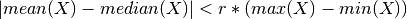Parameters: x (pandas.Series) – the time series to calculate the feature of r (float) – the percentage of the range to compare with the value of this feature bool

This function is of type: combiner

tsfresh.feature_extraction.feature_calculators.time_reversal_asymmetry_statistic(x, lag)[source]

This function calculates the value of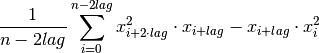which is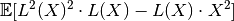whereis the mean andis the lag operator. It was proposed in  as a promising feature to extract from time series.

References

 Fulcher, B.D., Jones, N.S. (2014).
Highly comparative feature-based time-series classification.
Knowledge and Data Engineering, IEEE Transactions on 26, 3026–3037.
Parameters: x (pandas.Series) – the time series to calculate the feature of lag (int) – the lag that should be used in the calculation of the feature the value of this feature float

This function is of type: simple

tsfresh.feature_extraction.feature_calculators.value_count(x, value)[source]

Count occurrences of value in time series x.

Parameters: x (pandas.Series) – the time series to calculate the feature of value (int or float) – the value to be counted the count int

This function is of type: simple

tsfresh.feature_extraction.feature_calculators.variance(x)[source]

Returns the variance of x

Parameters: x (pandas.Series) – the time series to calculate the feature of the value of this feature float

This function is of type: simple

tsfresh.feature_extraction.feature_calculators.variance_larger_than_standard_deviation(x)[source]

Boolean variable denoting if the variance of x is greater than its standard deviation. Is equal to variance of x being larger than 1

Parameters: x (pandas.Series) – the time series to calculate the feature of the value of this feature bool

This function is of type: simple

## tsfresh.feature_extraction.settings module¶

This file contains methods/objects for controlling which features will be extracted when calling extract_features. For the naming of the features, see Feature Calculation.

class tsfresh.feature_extraction.settings.ComprehensiveFCParameters[source]

Bases: dict

Create a new ComprehensiveFCParameters instance. You have to pass this instance to the extract_feature instance.

It is basically a dictionary (and also based on one), which is a mapping from string (the same names that are in the feature_calculators.py file) to a list of dictionary of parameters, which should be used when the function with this name is called.

Only those strings (function names), that are keys in this dictionary, will be later used to extract features - so whenever you delete a key from this dict, you disable the calculation of this feature.

You can use the settings object with

>>> from tsfresh.feature_extraction import extract_features, ComprehensiveFCParameters
>>> extract_features(df, default_fc_parameters=ComprehensiveFCParameters())


to extract all features (which is the default nevertheless) or you change the ComprehensiveFCParameters object to other types (see below).

class tsfresh.feature_extraction.settings.EfficientFCParameters[source]

This class is a child class of the ComprehensiveFCParameters class and has the same functionality as its base class.

The only difference is, that the features with high computational costs are not calculated. Those are denoted by the attribute “high_comp_cost”.

You should use this object when calling the extract function, like so:

>>> from tsfresh.feature_extraction import extract_features, EfficientFCParameters
>>> extract_features(df, default_fc_parameters=EfficientFCParameters())

class tsfresh.feature_extraction.settings.MinimalFCParameters[source]

This class is a child class of the ComprehensiveFCParameters class and has the same functionality as its base class. The only difference is, that most of the feature calculators are disabled and only a small subset of calculators will be calculated at all. Those are donated by an attribute called “minimal”.

Use this class for quick tests of your setup before calculating all features which could take some time depending of your data set size.

You should use this object when calling the extract function, like so:

>>> from tsfresh.feature_extraction import extract_features, MinimalFCParameters
>>> extract_features(df, default_fc_parameters=MinimalFCParameters())

tsfresh.feature_extraction.settings.from_columns(columns)[source]

Creates a mapping from kind names to fc_parameters objects (which are itself mappings from feature calculators to settings) to extract only the features contained in the columns. To do so, for every feature name in columns this method

1. split the column name into col, feature, params part
2. decide which feature we are dealing with (aggregate with/without params or apply)
3. add it to the new name_to_function dict
4. set up the params
Parameters: columns (list of str) – containing the feature names The kind_to_fc_parameters object ready to be used in the extract_features function. dict

## Module contents¶

The tsfresh.feature_extraction` module contains methods to extract the features from the time series